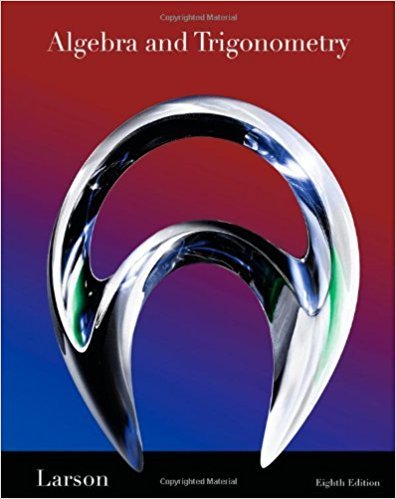×
Log in to StudySoup
Get Full Access to Math - Textbook Survival Guide
Join StudySoup for FREE
Get Full Access to Math - Textbook Survival Guide

Already have an account? Login here
×
Reset your password

Textbooks / Math / Algebra and Trigonometry 8

# Algebra and Trigonometry 8th Edition - Solutions by Chapter## Full solutions for Algebra and Trigonometry | 8th Edition

ISBN: 9781439048474Algebra and Trigonometry | 8th Edition - Solutions by Chapter

Solutions by Chapter
4 5 0 312 Reviews
##### ISBN: 9781439048474

Since problems from 83 chapters in Algebra and Trigonometry have been answered, more than 83208 students have viewed full step-by-step answer. The full step-by-step solution to problem in Algebra and Trigonometry were answered by , our top Math solution expert on 12/27/17, 07:37PM. This expansive textbook survival guide covers the following chapters: 83. Algebra and Trigonometry was written by and is associated to the ISBN: 9781439048474. This textbook survival guide was created for the textbook: Algebra and Trigonometry, edition: 8.

Key Math Terms and definitions covered in this textbook
• Cayley-Hamilton Theorem.

peA) = det(A - AI) has peA) = zero matrix.

• Complex conjugate

z = a - ib for any complex number z = a + ib. Then zz = Iz12.

• Ellipse (or ellipsoid) x T Ax = 1.

A must be positive definite; the axes of the ellipse are eigenvectors of A, with lengths 1/.JI. (For IIx II = 1 the vectors y = Ax lie on the ellipse IIA-1 yll2 = Y T(AAT)-1 Y = 1 displayed by eigshow; axis lengths ad

• Factorization

A = L U. If elimination takes A to U without row exchanges, then the lower triangular L with multipliers eij (and eii = 1) brings U back to A.

• Full row rank r = m.

Independent rows, at least one solution to Ax = b, column space is all of Rm. Full rank means full column rank or full row rank.

• Gauss-Jordan method.

Invert A by row operations on [A I] to reach [I A-I].

• Hessenberg matrix H.

Triangular matrix with one extra nonzero adjacent diagonal.

• Krylov subspace Kj(A, b).

The subspace spanned by b, Ab, ... , Aj-Ib. Numerical methods approximate A -I b by x j with residual b - Ax j in this subspace. A good basis for K j requires only multiplication by A at each step.

• lA-II = l/lAI and IATI = IAI.

The big formula for det(A) has a sum of n! terms, the cofactor formula uses determinants of size n - 1, volume of box = I det( A) I.

• Network.

A directed graph that has constants Cl, ... , Cm associated with the edges.

• Orthogonal matrix Q.

Square matrix with orthonormal columns, so QT = Q-l. Preserves length and angles, IIQxll = IIxll and (QX)T(Qy) = xTy. AlllAI = 1, with orthogonal eigenvectors. Examples: Rotation, reflection, permutation.

• Outer product uv T

= column times row = rank one matrix.

• Row space C (AT) = all combinations of rows of A.

Column vectors by convention.

• Singular matrix A.

A square matrix that has no inverse: det(A) = o.

• Singular Value Decomposition

(SVD) A = U:E VT = (orthogonal) ( diag)( orthogonal) First r columns of U and V are orthonormal bases of C (A) and C (AT), AVi = O'iUi with singular value O'i > O. Last columns are orthonormal bases of nullspaces.

• Special solutions to As = O.

One free variable is Si = 1, other free variables = o.

• Subspace S of V.

Any vector space inside V, including V and Z = {zero vector only}.

• Tridiagonal matrix T: tij = 0 if Ii - j I > 1.

T- 1 has rank 1 above and below diagonal.

• Unitary matrix UH = U T = U-I.

Orthonormal columns (complex analog of Q).

• Vector space V.

Set of vectors such that all combinations cv + d w remain within V. Eight required rules are given in Section 3.1 for scalars c, d and vectors v, w.